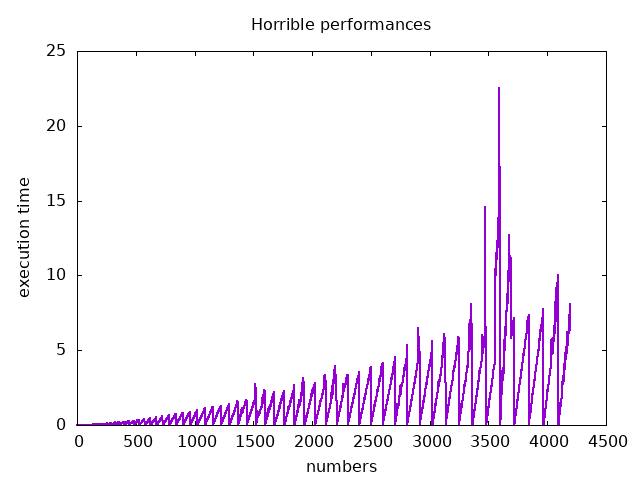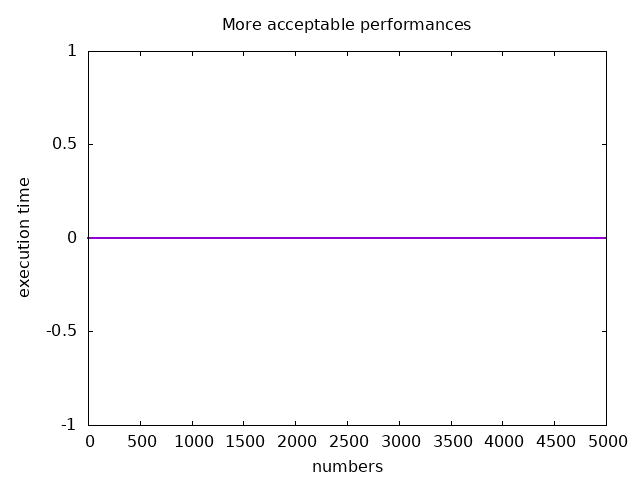# Calculating the square root of a number in pure shell

## Introduction

In order to write a raytracer in pure shell I need to be able to calculate the square root of any number in pure shell too, because using bc(1) is cheating.

As I do not have a particularly strong mathematical background I resorted to visit the Wikipedia article about calculating square roots and eliminated every algorithms too complicated for the poor old shell.

One important limitation to note is the total lack of floating-point arithmetic in this language: signed integers are the only “type” handled beside pure text. Fortunately this limitation is really easy to overcome using fixed-point arithmetic.

In all the examples underneath it is assumed that the scaling factor is 1000.

The code is hosted here if you do not want to read the details: https://git.sr.ht/~tleguern/krt/tree/master/item/sqrt.sh

## heron

The first algorithm I stumbled upon is named the “Babylonian method” or “Heron’s method”. It is apparently very old and for this reason alone I decided it was a good pick.

Here is it’s translation in POSIX shell:

``````heron() {
local S="\$1"; shift # Compute the non-negative square root of S,
local x="\$1"; shift # using x as a starting point.
if [ \$# -ge 1 ]; then
local maxsteps="\$1"
else
local maxsteps=5
fi
local steps=1

while [ "\$steps" -lt "\$maxsteps" ]; do
local nextx=\$(( (x + S * 1000 / x) / 2 ))
echo "-> \$nextx" >&2
if [ "\$x" = "\$nextx" ]; then
break
fi
x="\$nextx"
steps=\$(( steps + 1 ))
done
printf "%d\n" "\$x"
}
``````

There are two mandatory parameters: `S` is the number we want to calculate the square root of and `x` is a rough estimation to speed things off. An optional parameter `maxsteps` is also present because with enough precision it is apparently possible to search more or less indefinitely for a value. With this precaution the function stops if two consecutive runs yielded the same value or if the maximum number of steps is reached.

Fortunately the Wikipedia article includes an example of this algorithm at work using the following parameters: `S=125348` and `x=600`. Here is a rundown of my implementation preforming against these parameters:

``````\$ cat ./testsqrt.sh
#!/bin/sh

. ./sqrt.sh

S=125348
x=600
heron \$(( S * 1000 )) \$(( x * 1000))
\$ ./testsqrt.sh
-> 404456
-> 357186
-> 354059
-> 354045
Final result: 354045
``````

Without too much surprise using the same precision as the article it took the same number of steps to find a good enough answer: 354.045.

## bakhshali

The second interesting algorithm is the Bakhshali method who is just as old as `heron` but is less precise as it is hard-coded with only two steps. If the initial guess is not good enough the results are atrocious.

``````bakhshali() {
local S="\$1"; shift
local x="\$1"; shift

for steps in 1 2; do
local a=\$(( (S - x * x / 1000) * 1000 / (2 * x) ))
local b=\$(( x + a ))
local nextx=\$(( b - (a * a / 1000) * 1000 / (2 * b) ))
echo "-> \$nextx" >&2
x="\$nextx"
done
printf "%d\n" "\$x"
}
``````

Not much to say here.

## newton

My final implementation is Newton–Raphson method which I found somewhat similar to `heron`.

``````newton() {
local S="\$1"; shift
local x="\$1"; shift
if [ \$# -ge 1 ]; then
local maxsteps="\$1"
else
local maxsteps=5
fi
local steps=1

while [ "\$steps" -lt "\$maxsteps" ]; do
local nextx=\$(( x - (x * x / 1000 - S) * 1000 / (2 * x) ))
echo "-> \$nextx" >&2
if [ "\$x" = "\$nextx" ]; then
break
fi
x="\$nextx"
steps=\$(( steps + 1 ))
done
printf "%d\n" "\$x"
}
``````

I decided to it pick as my default implementation for now, because I needed to choose one and I do not have data justifying another decision.

## Finding a good first approximation

These three algorithms are all fine and yield nearly the same values all the time … if and only if the parameter `x` is good enough.

For example here are the best guesses of all three algorithms for the square root of 5 using a terrible initial estimate of 150:

• bakhshali: 9.555
• newton: 9.552
• heron: 9.551

Pushing the number of steps for `newton` and `heron` to 10 (remember that bakhshalimethod is hard coded with only two iterations) yields far more satisfying results:

• newton: 2.237
• heron: 2.236

So a good first approximation is crucial to reduce the number of steps and then in turn improve performances.

I wanted to explore the possibilities of initial estimations and implemented a simple brute force algorithm dedicated to dynamically finding the nearest smaller perfect square of a given `S`.

``````nearest() {
local x=0
local i="\$1"

while [ \$i -gt 0 ]; do
local j=1
while [ "\$j" -lt "\$i" ]; do
if [ \$((j * j)) -eq "\$i" ]; then
x="\$j"
break 2
fi
j=\$(( j + 1 ))
done
i=\$(( i - 1 ))
done
echo \$x
}
``````

This is a pure brute force solution making it perfectly reasonable for any decent programming languages, meaning it excludes the shell.

Here is a performance graph:I initially wanted to graph all the way to 5000 but got bored before the end. The big spike around 3500 is unrelated and caused by activity on my laptop.

This calculation become slow very quickly: the first number whose nearest square is found in more than one second is 1084, more than two seconds is 1519 and three is 1925. These are not particularly big numbers.

To reduce this performance cost I decided to use a pre-computed list of squares and to just perform look up when needed. Assuming this list is named `perfect_squares.txt` here is a faster implementation:

``````nearest() {
local x=1
local i="\$1"

local input=./perfect_squares.txt
# Push the space separated list of numbers as positionnal parameters
set \$(< \$input)
while ! [ "\$i" -le "\$2" ]; do
x=\$(( x + 1 ))
shift # Shift the list forward, let the shell do the hard work
done
echo "\$x"
}
``````

Using the built-in utility `set` I push the content of `./perfect_squares.txt` as positional parameters, meaning each word is accessible as `\$1`, `\$2`, etc… During each iteration if the square currently compared is not the right one I `shift` it and move along.

The performance graph for this implementation is boring:## Cheating

And what about skipping all this and using `bc(1)` instead ? Easy:

``````cheating_with_bc() {
local S="\$1"; shift
local factor="\${FACTOR:-1000}"
local scale="\$(printf "%d" \$factor | tr -d '[1-9]' | wc -c)"

local res="\$(bc -l -e "scale=\$scale; sqrt(\$S/\$factor)*\$factor" -e quit)"
echo "\$res" | cut -d '.' -f 1
}
``````

While the execution time is just as constant as with the pre-computed method the additional pipes are an unwanted source of slowness for later scripts.

## Changelog

• 2021-01-14: Initial write-up ;
• 2021-01-21: Add the § Cheating section and link to shell-fixedpoint ;